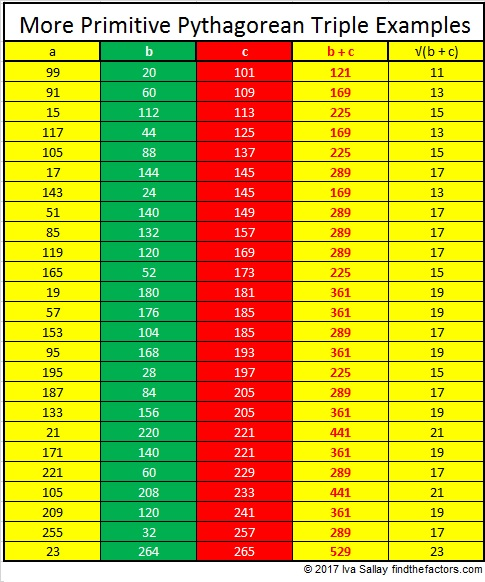# 821 Why Do Primitive Pythagorean Triples Do That?

The most famous Pythagorean triple is 3-4-5.

Perhaps you know that of the three numbers in EVERY Pythagorean triple at least one of them will be divisible by 3, at least one of them will be divisible by 4, and at least one of them will be divisible by 5. That’s obvious for triple 3-4-5.

Here’s another example: Pythagorean triple 11-60-61. Two of those numbers are prime numbers, yet 60 is divisible by 3, 4, AND 5.

If the Pythagorean triple is a primitive, something else amazing happens:That’s amazing all by itself, but that’s only part of the picture. Let’s look at the complete picture specifically using the triple with hypotenuse 821:

• 25² + 14² = 821
• 429² + 700² = 821²
• 429700821 can be calculated from 25² – 14², 2(25)(14), 25² + 14²
• 821 + 700 = 1521
• √1521 = 39 which is 25 + 14
• 429 ÷ 39 = 11

WHY does this happen to primitive Pythagorean triples?

It happened for 429700821 because 700 82125² + 14² + 2(25)(14) = (25 + 14)².

After all (a + b)² = a² +2ab + b² is always true.

And it can be rearranged: (a + b)² = a² + b² +2ab.

You can use a similar proof whenever the element of the Primitive triple that is divisible by 4 can be expressed as 2ab.

************

But it is a different, and perhaps simpler, story for many triples such as 94041 which was calculated using (9)(1), (9² – 1²)/2, (9² + 1²)/2.

In that case 40 + 41(9² – 1²)/2 + (9² + 1²)/2 = (9² – 1² + 9² + 1²)/2 = 9².

You can use a similar proof whenever the element of the Primitive triple that is divisible by 4 can be expressed as (a² – b²)/2.

************

Go ahead, take ANY primitive Pythagorean triple. Add the leg that is divisible by 4 to its hypotenuse. You will get a perfect square. Here’s a few more examples:Similarly primitive triples that have been multiplied by a square number will also produce a perfect square, but you’ll have to be careful which leg you add to the hypotenuse if you multiplied by a square number that is a multiple of 4.

For example, 9(3-4-5) = 27-36-45 and 36 + 45 = 81, a square number.

But 4(3-4-5) = 12-16-20 so each number is divisible by 4. Note that 16 + 20 = 36, a square number, but 12 + 20 does not.

Here’s a few other essential facts about the number 821:

All of the odd numbers between 820 and 830, except 825, are prime numbers. Thus 821, 823, 827, 829 is the fourth prime decade.

• 821 is a prime number.
• Prime factorization: 821 is prime.
• The exponent of prime number 821 is 1. Adding 1 to that exponent we get (1 + 1) = 2. Therefore 821 has exactly 2 factors.
• Factors of 821: 1, 821
• Factor pairs: 821 = 1 x 821
• 821 has no square factors that allow its square root to be simplified. √821 ≈ 28.65309756.

How do we know that 821 is a prime number? If 821 were not a prime number, then it would be divisible by at least one prime number less than or equal to √821 ≈ 28.7. Since 821 cannot be divided evenly by 2, 3, 5, 7, 11, 13, 17, 19, or 23, we know that 821 is a prime number.Here’s another way we know that 821 is a prime number: Since its last two digits divided by 4 leave a remainder of 1, and 25² + 14² = 821 with 25 and 14 having no common prime factors, 821 will be prime unless it is divisible by a prime number Pythagorean triple hypotenuse less than or equal to √821 ≈ 28.7. Since 821 is not divisible by 5, 13, or 17, we know that 821 is a prime number.